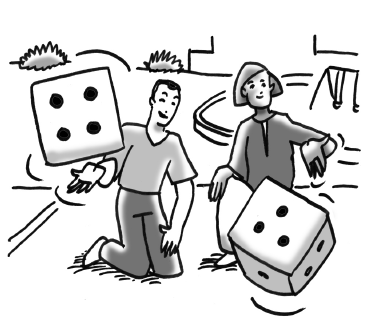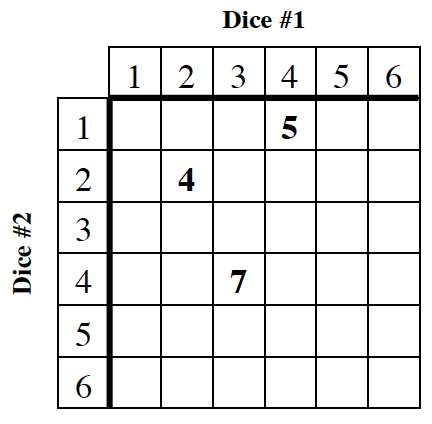Home > INT2 > Chapter 3 > Lesson 3.1.1 > Problem3-6

3-6.

ROLL AND WINYou begin the game Roll and Win by picking a number. Then you roll two regular dice, each numbered $1$ through $6$, and add the numbers that come up together. If the sum is the number you chose, you win a point. For example, if you choose “$11$”, and a $6$ and a $5$ are rolled, you win! 3-6 HW eTool (CPM)   Homework Help ✎

1. What is the sample space, which can be thought of as the set of all the possible outcomes, when two dice are rolled and their numbers added?

What sums can occur when two dice are rolled?

2. One way to analyze this situation is to make a table of all the possible outcomes like the one below. Copy and complete this table of sums on your paper. Are each of the outcomes in this table equally likely?

Is one number on a die more likely to come up than another?

3. What is $P(\text{even})$? $P(10)$? $P(15)$?

Use your completed table to answer this part.

4. Which sum is the most likely result? What is the probability of rolling that sum?

Which number appears most often in the table?

$\text{P}(7) = \frac{6}{36} = \frac{1}{6}$

Use the eTool below to complete the table.
Click the link at right for the full version of the eTool: INT2 3-6 HW eTool